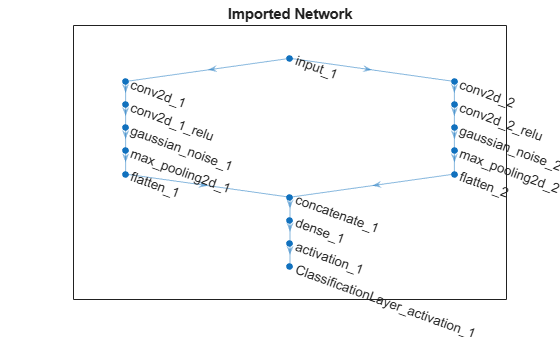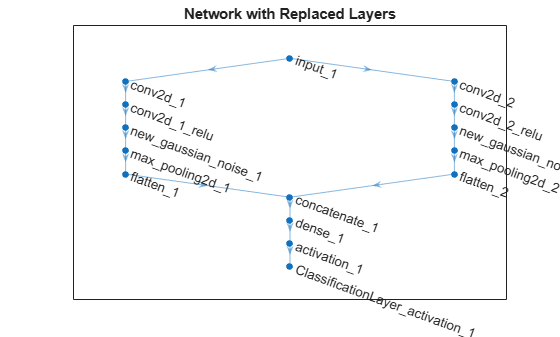# replaceLayer

Replace layer in layer graph

## Syntax

``newlgraph = replaceLayer(lgraph,layerName,larray)``
``newlgraph = replaceLayer(lgraph,layerName,larray,'ReconnectBy',mode)``

## Description

example

````newlgraph = replaceLayer(lgraph,layerName,larray)` replaces the layer `layerName` in the layer graph `lgraph` with the layers in `larray`. `replaceLayer` connects the layers in `larray` sequentially and connects `larray` into the layer graph.```

example

````newlgraph = replaceLayer(lgraph,layerName,larray,'ReconnectBy',mode)` additionally specifies the method of reconnecting layers.```

## Examples

collapse all

Define a simple network architecture and plot it.

```layers = [ imageInputLayer([28 28 1],'Name','input') convolution2dLayer(3,16,'Padding','same','Name','conv_1') reluLayer('Name','relu_1') additionLayer(2,'Name','add') fullyConnectedLayer(10,'Name','fc') softmaxLayer('Name','softmax') classificationLayer('Name','classoutput')]; lgraph = layerGraph(layers); lgraph = connectLayers(lgraph,'input','add/in2'); figure plot(lgraph)```Replace the ReLU layer in the network with a batch normalization layer followed by a leaky ReLU layer.

```larray = [batchNormalizationLayer('Name','BN1') leakyReluLayer('Name','leakyRelu_1','Scale',0.1)]; lgraph = replaceLayer(lgraph,'relu_1',larray); plot(lgraph)```This example shows how to import the layers from a pretrained Keras network, replace the unsupported layers with custom layers, and assemble the layers into a network ready for prediction.

Import Keras Network

Import the layers from a Keras network model. The network in `'digitsDAGnetwithnoise.h5'` classifies images of digits.

```filename = 'digitsDAGnetwithnoise.h5'; lgraph = importKerasLayers(filename,'ImportWeights',true);```
```Warning: Unable to import some Keras layers, because they are not supported by the Deep Learning Toolbox. They have been replaced by placeholder layers. To find these layers, call the function findPlaceholderLayers on the returned object. ```

The Keras network contains some layers that are not supported by Deep Learning Toolbox. The `importKerasLayers` function displays a warning and replaces the unsupported layers with placeholder layers.

Plot the layer graph using `plot`.

```figure plot(lgraph) title("Imported Network")```Replace Placeholder Layers

To replace the placeholder layers, first identify the names of the layers to replace. Find the placeholder layers using `findPlaceholderLayers`.

`placeholderLayers = findPlaceholderLayers(lgraph)`
```placeholderLayers = 2x1 PlaceholderLayer array with layers: 1 'gaussian_noise_1' PLACEHOLDER LAYER Placeholder for 'GaussianNoise' Keras layer 2 'gaussian_noise_2' PLACEHOLDER LAYER Placeholder for 'GaussianNoise' Keras layer ```

Display the Keras configurations of these layers.

`placeholderLayers.KerasConfiguration`
```ans = struct with fields: trainable: 1 name: 'gaussian_noise_1' stddev: 1.5000 ```
```ans = struct with fields: trainable: 1 name: 'gaussian_noise_2' stddev: 0.7000 ```

Define a custom Gaussian noise layer. To create this layer, save the file `gaussianNoiseLayer.m` in the current folder. Then, create two Gaussian noise layers with the same configurations as the imported Keras layers.

```gnLayer1 = gaussianNoiseLayer(1.5,'new_gaussian_noise_1'); gnLayer2 = gaussianNoiseLayer(0.7,'new_gaussian_noise_2');```

Replace the placeholder layers with the custom layers using `replaceLayer`.

```lgraph = replaceLayer(lgraph,'gaussian_noise_1',gnLayer1); lgraph = replaceLayer(lgraph,'gaussian_noise_2',gnLayer2);```

Plot the updated layer graph using `plot`.

```figure plot(lgraph) title("Network with Replaced Layers")```Specify Class Names

If the imported classification layer does not contain the classes, then you must specify these before prediction. If you do not specify the classes, then the software automatically sets the classes to `1`, `2`, ..., `N`, where `N` is the number of classes.

Find the index of the classification layer by viewing the `Layers` property of the layer graph.

`lgraph.Layers`
```ans = 15x1 Layer array with layers: 1 'input_1' Image Input 28x28x1 images 2 'conv2d_1' Convolution 20 7x7x1 convolutions with stride [1 1] and padding 'same' 3 'conv2d_1_relu' ReLU ReLU 4 'conv2d_2' Convolution 20 3x3x1 convolutions with stride [1 1] and padding 'same' 5 'conv2d_2_relu' ReLU ReLU 6 'new_gaussian_noise_1' Gaussian Noise Gaussian noise with standard deviation 1.5 7 'new_gaussian_noise_2' Gaussian Noise Gaussian noise with standard deviation 0.7 8 'max_pooling2d_1' Max Pooling 2x2 max pooling with stride [2 2] and padding 'same' 9 'max_pooling2d_2' Max Pooling 2x2 max pooling with stride [2 2] and padding 'same' 10 'flatten_1' Keras Flatten Flatten activations into 1-D assuming C-style (row-major) order 11 'flatten_2' Keras Flatten Flatten activations into 1-D assuming C-style (row-major) order 12 'concatenate_1' Depth concatenation Depth concatenation of 2 inputs 13 'dense_1' Fully Connected 10 fully connected layer 14 'activation_1' Softmax softmax 15 'ClassificationLayer_activation_1' Classification Output crossentropyex ```

The classification layer has the name `'ClassificationLayer_activation_1'`. View the classification layer and check the `Classes` property.

`cLayer = lgraph.Layers(end)`
```cLayer = ClassificationOutputLayer with properties: Name: 'ClassificationLayer_activation_1' Classes: 'auto' ClassWeights: 'none' OutputSize: 'auto' Hyperparameters LossFunction: 'crossentropyex' ```

Because the `Classes` property of the layer is `'auto'`, you must specify the classes manually. Set the classes to `0`, `1`, ..., `9`, and then replace the imported classification layer with the new one.

`cLayer.Classes = string(0:9)`
```cLayer = ClassificationOutputLayer with properties: Name: 'ClassificationLayer_activation_1' Classes: [0 1 2 3 4 5 6 7 8 9] ClassWeights: 'none' OutputSize: 10 Hyperparameters LossFunction: 'crossentropyex' ```
`lgraph = replaceLayer(lgraph,'ClassificationLayer_activation_1',cLayer);`

Assemble Network

Assemble the layer graph using `assembleNetwork`. The function returns a `DAGNetwork` object that is ready to use for prediction.

`net = assembleNetwork(lgraph)`
```net = DAGNetwork with properties: Layers: [15x1 nnet.cnn.layer.Layer] Connections: [15x2 table] InputNames: {'input_1'} OutputNames: {'ClassificationLayer_activation_1'} ```

## Input Arguments

collapse all

Layer graph, specified as a `LayerGraph` object. To create a layer graph, use `layerGraph`.

Name of the layer to replace, specified as a string scalar or a character vector.

Network layers, specified as a `Layer` array.

For a list of built-in layers, see List of Deep Learning Layers.

Method to reconnect layers specified as one of the following:

• `'name'` – Reconnect `larray` using the input and output names of the replaced layer. For each layer connected to an input of the replaced layer, reconnect the layer to the input of the same input name of `larray(1)`. For each layer connected to an output of the replaced layer, reconnect the layer to the output of the same output name of `larray(end)`.

• `'order'` – Reconnect `larray` using the order of the input names of `larray(1)` and the output names of `larray(end)`. Reconnect the layer connected to the `i`th input of the replaced layer to the `i`th input of `larray(1)`. Reconnect the layer connected to the `j`th output of the replaced layer to the `j`th output of `larray(end)`.

Data Types: `char` | `string`

## Output Arguments

collapse all

Output layer graph, returned as a `LayerGraph` object.

## Version History

Introduced in R2018b